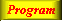Lecture 3 - Page 17 : 42
 Functional Programming in SchemeName binding, Recursion, Iteration, and Continuations * Name binding constructs The let name binding expression The equivalent meaning of let Examples with let name binding The let* name binding construct An example with let* The letrec namebinding construct LAML time functions * Conditional expressions Conditional expressions Examples with if Example with cond: leap-year? Example with cond: american-time Example with cond: as-string * Recursion and iteration Recursion List processing Tree processing (1) Tree processing (2) Recursion versus iteration Example of recursion: number-interval Examples of recursion: string-merge Examples with recursion: string-of-char-list? Exercises * Example of recursion: Hilbert Curves Hilbert Curves Building Hilbert Curves of order 1 Building Hilbert Curves of order 2 Building Hilbert Curves of order 3 Building Hilbert Curves of order 4 A program making Hilbert Curves * Continuations Introduction and motivation The catch and throw idea A catch and throw example The intuition behind continuations Being more precise The capturing of continuations Capturing, storing, and applying continuations Use of continuations for escaping purposes Practical example: Length of an improper list Practical example: Searching a binary tree
 List processing
 A list is a recursive data structure As a consequence list processing is done via recursive functions We illustrate list processing by extracting attribute values from a LAML attribute property list
 ```; Return the href attribute value from a property list ; Return #f if no href attribute is found. ; Pre-condition: attr-p-list is a property list - ; of even length. (define (find-href-attribute attr-p-list) (if (null? attr-p-list) #f (let ((attr-name (car attr-p-list)) (attr-value (cadr attr-p-list)) (attr-rest (cddr attr-p-list))) (if (eq? attr-name 'href) attr-value (find-href-attribute attr-rest)))))```

A function for extraction of the href attribute from a property list.An example with property lists that represent HTML attributes in LAML.

As the last interaction, we see the function find-href-attribute in play.